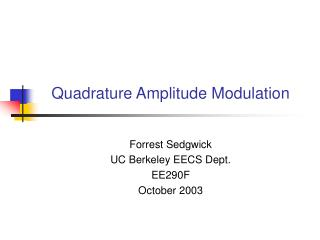DownloadDownload PresentationTélécharger la présentation- - - - - - - - - - - - - - - - - - - - - - - - - - - E N D - - - - - - - - - - - - - - - - - - - - - - - - - - -
##### Presentation Transcript

1. Quadrature Amplitude Modulation Forrest Sedgwick UC Berkeley EECS Dept. EE290F October 2003

2. Analog vs Digital • Information Theory vs Signal Analysis • Discrete Levels vs Analogous Representation • Sacrifice arbitrarily precise representation of signal • Gain arbitrary degree of reproducibility of given signal • KEY BENEFIT • Discrete information can be transmitted with arbitrarily low error rates EVEN ON A NOISY CHANNEL • Digital information content measured in units of bits, decimals, or nats

3. Shannon’s Channel Capacity • Channel capacity C (bits/sec) is the speed at which information can travel over a channel with an arbitrarily low error rate i.e. when a system is transmitting bits at or below C then for any BER e>0 there exists a code with block length n which will provide a BER < e. www-gap.dcs.st-and.ac.uk/~history/ Mathematicians/Shannon.html Assumes noise is thermal – Gaussian and White

4. Modulation • All channels consist of some continuous parameter • Must map discrete states onto continuous property • Must have a decision circuit to map the state of the modulated channel into a discrete state • As number of levels or states M the behavior of the digital system does not approach that of an analog system, due to the decision circuit

5. Number of Levels • Digital communications relies on a finite number of discrete levels • Minimum number of levels is two (binary code) • Shannon Capacity helps determine optimum number of levels for a given bandwidth, SNR, and BER

6. Limits on Communication Channels • Two types of communication channels • r<<1 – Power Limited • High dimensionality signaling schemes • Binary • r>>1 – Bandwidth Limited • Low dimensionality • Multilevel Proakis and Salehi, pp. 738

7. Modulation Scheme • A channel with lowpass frequency characteristics is called baseband. Digital information is transmitted directly • Ex. Pulse Amplitude Modulation (PAM) • A channel far removed from DC (like optical) is called a bandpass channel • Transmission on a bandpass channel requires modulation of a carrier • Amplitude Shift Keying (ASK) • Phase Shift Keying (PSK) • Frequency Shift Keying (FSK • Quadrature Amplitude Modulation (QAM)

8. Amplitude Shift Keying (ASK) • Amplitude of carrier wave is modulated • Equivalent BER vs SNR to baseband PAM Proakis and Salehi, pp. 306

9. Angle Modulation (PSK and FSK) • Frequency is time derivative of phase, PSK and FSK are somewhat equivalent Proakis and Salehi, pp. 332

10. PSK: Digital Angle Modulation • Usually in digital communications PSK is chosen over FSK • Easier to create multilevel codes • Possibility of using differential phase shift keying (DPSK) • Uses phase shifts relative to previous bit • Eliminates need for local oscillator at receiver • Use Gray Code to minimize effect of errors Proakis and Salehi, pp. 631

11. Quadrature Amplitude Modulation • Amplitude and Phase of carrier are modulated • Discrete amplitudes and phases form a constellation • Can also think of QAM as a “complex” amplitude modulation scheme Proakis and Salehi, pp. 653

12. Constellations • Different constellations require different SNR for a given BER • (d) is lowest power by about 1dB (for given BER) • (a) and (b) are rectangular • Rectangular constellations offer very simple modulation/ demodulation schemes • ASK two quadrature carriers - same frequency but 90 out of phase • Mix quadrature carriers for output Proakis and Salehi, pp. 653

13. QAM vs ASK (multilevel) • QAM has a tremendous advantage in noise performance • Energy in every bit (including zero) • Substantially more complex (coherent detection vs photodiode) Proakis and Salehi, pp. 565 Proakis and Salehi, pp. 495

14. QAM vs PSK • 4-QAM and 4-PSK have same power penalty • For k>4, k-QAM is an improvement over k-PSK Proakis and Salehi, pp. 639

15. Applications of QAM • Used in bandwidth-limited applications • Modems: telephones have 3kHz bandwidth, excellent SNR (20dB) => M-ary QAM • Cellular Telephones: Bandwidth is at a premium, very expensive (However, POWER is also at a premium...)

16. Limitations • Almost always requires a highly stable local oscillator • In the optical domain this is very expensive • Possible (but difficult) to use differential phase keying • Performance limits still not reached for • Direct detection • Signal Dimensionality (DWDM) • Transmitter Power

17. References • John G. Proakis, Masoud Salehi, Communications Systems Engineering, Prentice Hall 1994